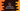# C++ how to find the smallest of three numbers## How to find the smallest of three numbers in C++:

In this post, we will learn how to find the smallest of three given numbers in C++. We can solve it in different ways. The easiest way to solve it is by comparing each numbers with one another. But we can also write it in an efficient way.

### Method 1: Find the smallest of three numbers by comparing with one another:

In this method, we will learn how to find the smallest of three numbers by comparing the numbers with one another. This program will read all numbers from the user and print out the smallest of all:

``````#include <iostream>
using namespace std;

int findSmallest(int first, int second, int third)
{
if (first <= second && first <= third)
{
return first;
}
else if (second <= first && second <= third)
{
return second;
}
else
{
return third;
}
}

int main()
{
int first, second, third;

cout << "Enter the first number :" << endl;
cin >> first;

cout << "Enter the second number :" << endl;
cin >> second;

cout << "Enter the third number :" << endl;
cin >> third;

cout << "Smallest value : " << findSmallest(first, second, third) << endl;
}``````

Here,

• we are reading the numbers and storing them in the variables first, second and third.
• findSmallest method is used to find the smallest of the three user input numbers.
• It compares the first and the second number with the others and based on that returns the smallest.

It will print output as like below:

``````Enter the first number :
4
Enter the second number :
2
Enter the third number :
3
Smallest value : 2``````

### Method 2: Finding the smallest number in one line:

We can use std::min to find the minimum of three values as like below:

``````#include <iostream>
using namespace std;

int findSmallest(int first, int second, int third)
{
return min(first, min(second, third));
}

int main()
{
int first, second, third;

cout << "Enter the first number :" << endl;
cin >> first;

cout << "Enter the second number :" << endl;
cin >> second;

cout << "Enter the third number :" << endl;
cin >> third;

cout << "Smallest value : " << findSmallest(first, second, third) << endl;
}``````

Here, the outer min is finding the minimum value between first and minimum of second and third. So, it is actually finding the minimum value of the three values.

It will print similar output as the above program.